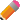## Introduction to Formula Basic, Cube Formulas, Dependencies & Persistent CalculationsThe succeeding section continues a discussion of formulas, which extend your ability to calculate cell values far beyond the simple summations and weight assignments that define Aggregate members within Dimension hierarchies. With Cube formulas, you can create more complex relationships between variables to solve real-world business problems. You are able to use standard pre-built mathematical expressions, or formulas you build yourself, to define values in single cells, across a range of cells, or even between cells in different Cubes within a database.

PowerOLAP® provides extended support, through dialog features and through formula "grammar," for arithmetic calculations, functions, and algebraic expressions. The enhanced Formula Editor has features that can be accessed by right-clicking in the dialog box. These features are explained under the topics Cube Formulas, Calculation Depth and Persistent Calculations.

In addition, PowerOLAP® allows you to write Dependencies for your formulas, greatly increasing the speed of calculations in large and sparsely populated databases. See the Dependencies topic for a more detailed explanation.

Persistent Calculation results are written to disk as opposed to existing solely in RAM, and provide a speed enhancement because these formulas do not calculate each time the Cube is recalculated. For a more in-depth understanding refer to the discussion on Persistent Calculation at the end of this section.NotesIn the succeeding topics, the Avon Trading Database located in the C:\Program Files (x86)\PowerOLAP\Examples directory is used.